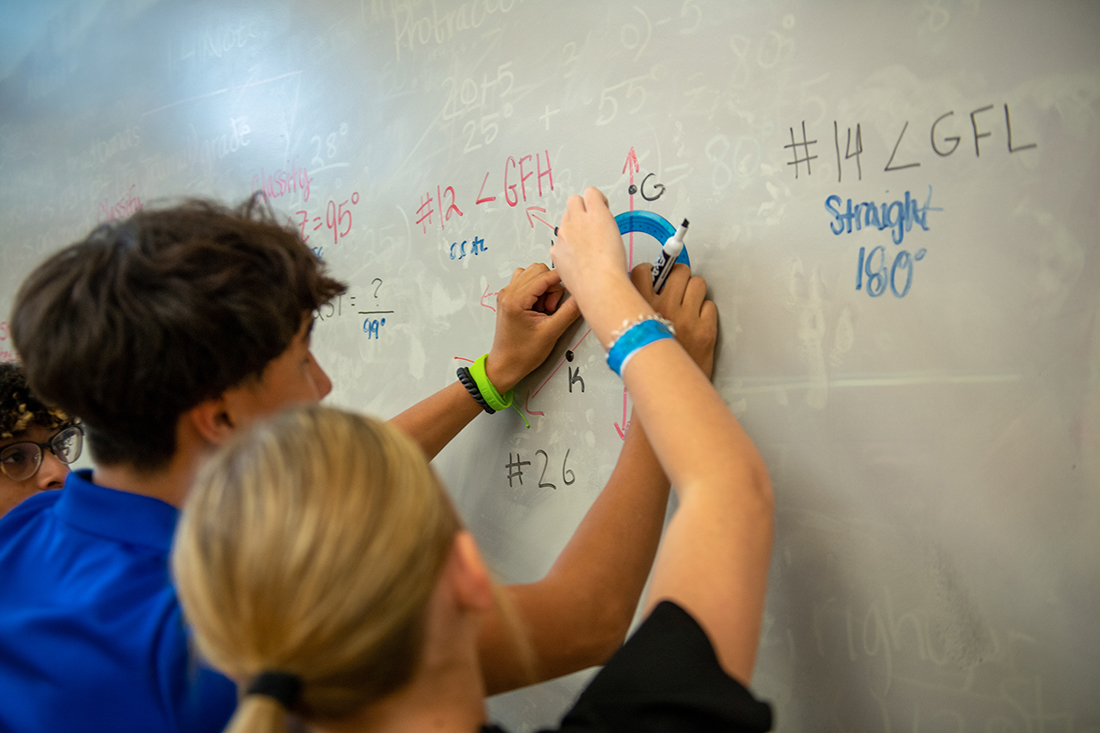Mathematics is a way of thinking. It is also a language in itself, a way of expressing ideas that lends itself to solving problems. In teaching mathematics, students are developing this way of thinking to help them become proficient in expressing ideas in the language of mathematics.

Faculty Members
Mr. Benjamin Boyle
Mr. Jim Jenkins
Mrs. Sarah Ramsey
Ms. Kimberly Tomasek

Algebra I
1 Credit ~ 2 Semesters ~ Prerequisite: None
This is the traditional starting point in high school math sequence. The skills developed in this course include: simplifying variable expressions, solving equations, graphing and problem solving. Most of the course will work in the rational number field, but students will be exposed to simplifying radicals and solving equations with irrational roots.

Algebra I Honors
1 Credit ~ 2 Semesters ~ Prerequisites: Previous academic math grades of 94 or above, and 90 percentile in standardized math test
The objectives of this section includes all the objectives of the regular Algebra I course, but the students will be exposed to more aspects of the theory and to more challenging problems.

Geometry
1 Credit ~ 2 Semesters ~ Prerequisite: Algebra I
This course covers the concepts of Plane Geometry and extends these concepts to 3-dimensional situations where possible. The first semester includes formal proofs. In second semester calculations are emphasized with formally proven theorems as part of the explanation.

Geometry Honors
1 Credit ~ 2 Semesters ~ Prerequisites: Algebra I and fulfillment of the honors program
The objectives of this course include all the objectives of the regular Geometry course with more emphasis on formal proofs and more challenging problems. Knowing the formulas is assumed and given information will not be the exact dimensions needed for the formulas. The challenge is to use the given information to calculate the dimensions needed to compute the area or volume.

Algebra II
1 Credit ~ 2 Semesters ~ Prerequisite: Geometry or concurrent enrollment in geometry
The objectives of this course are to reinforce the ideas of Algebra I and to extend them further develop the skill levels in the basic operations of Algebra, and to apply these skills to the Complex Number Field and higher degree polynomials.

Algebra II Honors
1 Credit ~ 2 Semesters ~ Prerequisite: Geometry or concurrent enrollment in Geometry and fulfillment of honors program requirements The objectives of this course are basically the same as Algebra II, with more emphasis on formally developing the ideas and applying the basic skills of Algebra to more challenging problems.

Algebra III
1 Credit ~ 2 semesters ~ Prerequisite: Algebra II
This course is designed as an alternative to Pre-Cal on the senior level. It bridges the gap in Algebra from Algebra II to College Algebra. Emphasis will be placed on enhancing Algebra skills. Some students may qualify for dual credit with VC in College Algebra.

Pre-Calculus 1
Credit ~ 2 Semesters ~ Prerequisite: Algebra II
This course extends the ideas and skills of Algebra and introduces some concepts of Calculus. Recognition of patterns, calculator skills, and an analytic approach to problem solving are emphasized. The course also covers all the basic concepts of Trigonometry.

Pre-Calculus Honors
1 Credit ~ 2 Semesters ~ Prerequisites: Algebra II and fulfillment of honors program requirements
The objectives of this course are basically the same as the regular sections of Pre-Calculus. In this course emphasis is placed on a student successfully solving more complex problems and handling the subtleties of the concepts.

Calculus
1 Credit ~ 2 Semesters ~ Prerequisite: Pre-Calculus and fulfillment of both AP and VC requirements.
This course follows the syllabus provided by both College Board AP Calculus AB and Victoria College Calculus I. Topics covered include Analysis of Function and their graphs, Limits of Functions, Derivatives, Application of Derivatives, Integrals and Application of Integrals.

Discrete Math and Logic
1 Credit ~ 2 Semesters ~ Prerequisite: Grade 11 and 12
Discrete math explores distinct, countable elements and structures present in the world. Instead of analyzing the continuous ideas present in mathematical fields such as algebra and calculus, discrete math examines individual elements and statements to either prove or disprove their truth value.  This is a math course that highlights logical thinking over repetitive computation.  Algebra 2 completion required as a prerequisite.  Algebra 2 concurrent enrollment considered on a case by case basis.

Statistics
1 Credit ~ 2 Semesters ~ Prerequisite: Grade 11 and 12
This is an introductory course to the study of statistics and probability and will provide students with the basic concepts of data analysis and statistical computing.  Students will learn to interpret the numbers in surveys, election polls, sports, and medical studies.  They will use graphs such as histograms, stem plots, time plots, and scatter plots to display data, using numbers such as median, mean, and standard deviation to describe data, evaluate data distribution, and calculate probability of events.  Topics include sampling, surveys, experimental design, organizing data, distributions, probability and inference.